# Ellipse

Ellipse is an important topic for the JEE exam. Ellipse is the set of all points on a plane whose distance from two points add up to a constant. In this article, we come across definition, ellipse equation and properties which helps students to have a deep understanding about the topic. Some of the most important equations of an ellipse include area and circumference, tangent equation, the tangent equation in slope form, chord equation, normal equation and the equation of chord joining the points of the ellipse.

## What is Ellipse?

An ellipse is a locus of a point which moves in such a way that its distance from a fixed point (focus) to its perpendicular distance from a fixed straight line (directrix) is constant. i.e. eccentricity(e) which is less than unity.

(0 < e < 1)

#### Definitions of an Ellipse### Standard Equation of Ellipse

The standard equation of an ellipse is given as:

(x2 / a2) + (y2 / b2) = 1  For, a>b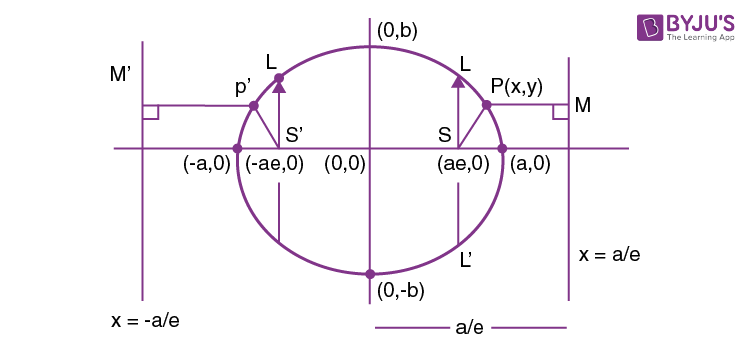So, from definition

[(SP) / (PM)] = e < 1 where P(x,y) is variable point.

(x − ae)2 + (y − 0)2 = e2 [(x – a/e) / 1]2

$$\begin{array}{l}\frac{x^{2}}{a^{2}}+\frac{y^{2}}{a^{2}(1-e^{2})}= 1\end{array}$$

⇒ x2 (1 – e2) + y2 = a2 (1 – e2)

Comparing both equation

b2 = a2 (1 – e2) e2 = [(a2 – b2) / a2]

Where a ⇒ Semi major axis.

b ⇒ Semi minor axis.

### Latus Rectum of an Ellipse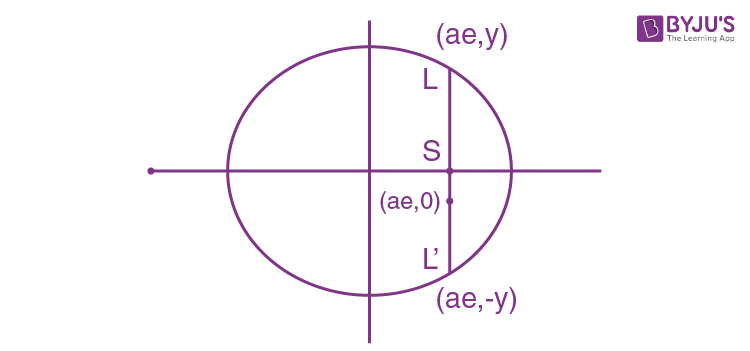Chord LSL’ is called Latus rectum

(x2 / a2 + y2 / b2)= 1, Substitute (x = ae)

(a2 e2 / a2) +  (y2 / b2) = y2 = b2 (1 – e2) y2 = (b4 / a2) y = ± (b2 / a) ⇒ (b2 / a), (−b2 / a)

Length of Latus Rectum = 2b2/a

 Important Points to Remember: Length of major axis = 2a Length of minor axis = 2b Equation of directrix, x = + a/e Length of Latus Rectum = (2b2 / a) Circle is a particular case of ellipse, where e = 0 C (0, 0) is centre of ellipse.

#### Area of an ellipse

The area of an ellipse = πab, where a is the semi major axis and b is the semi minor axis.

#### Circumference of an ellipse

The approximate value of the circumference of ellipse could be calculated as:

$$\begin{array}{l}L = \pi \sqrt{2(a^{2}+b^{2})}\end{array}$$

#### Position of point related to Ellipse

Let the point p(x1, y1) and ellipse

(x2 / a2) + (y2 / b2) = 1

If [(x12 / a2)+ (y12 / b2) − 1)]

= 0 {on the curve}

<0{inside the curve}

>0 {outside the curve}

Parametric form of equation of ellipse:

x = a cos Ɵ

y = b sin Ɵ

[(x2 / a2 )+ (y2 / b2)] = 1

### Auxiliary Circle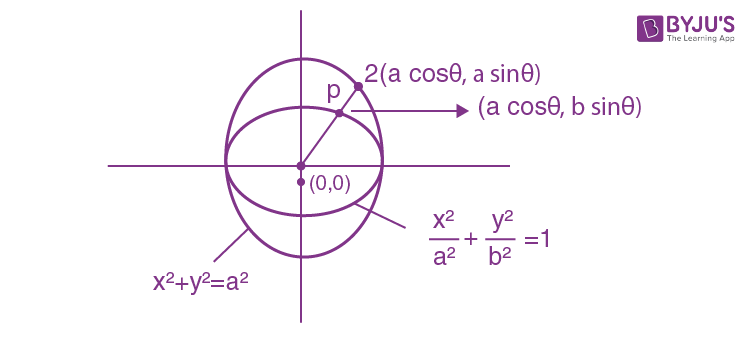Recommended Video### Some Important Equations of Ellipse

Some of the most important equations of an ellipse include tangent, the tangent equation in slope form, chord equation, normal equation and the equation of chord joining the points of the ellipse. All these equation are explained below in detail.

#### Tangent of ellipse

The tangent of an ellipse is a line that touches a point on the curve of the ellipse.

Let the equation of ellipse be [(x2 / a2) + (y2 /  b2)] = 1

the slope at point p(x1, y1)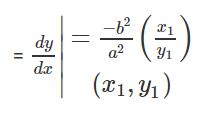#### Equation of Tangent

y – y1 = [(− b2 x1 ) / (a2 y1)] (x – x1)

⇒ (xx1 / a2 ) + (yy1 / b2) = 1, point form

⇒at point (acosθ, bsinθ), equation of tangent is (xcosθ / a) + (ysinθ / b) = 1

⇒ Tangent in slope form y = mx + c

Where,

$$\begin{array}{l}C = \pm \sqrt{a^{2}m^{2}+b^{2}}\end{array}$$

$$\begin{array}{l}\Rightarrow y = mx\pm \sqrt{a^{2}m^{2}+b^{2}}\end{array}$$

#### Equation of Tangent in slope form

Tangent from an external point p(x1, y1) to the ellipse

(x2 / a2) + (y2 / b2) = 1.

SS1  = T2

S ≡ (x2 / a2) + (y2 / b2) −1=0

S1 ≡ [(x12 / a2) + (y12 / b2)] – 1 = 0

T ≡ [(xx1 / a2) + (yy1 / b2)] – 1 = 0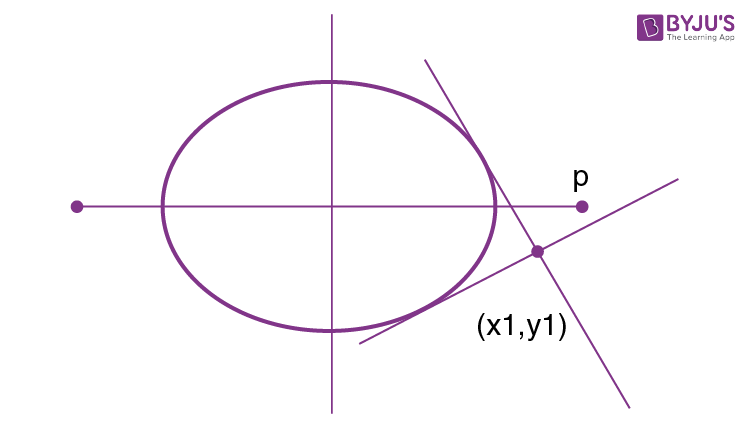#### Equation of chord with mid point (x1, y1)

The chord of an ellipse is a straight line which passes through two points on the ellipse’s curve. The chord equation of an ellipse having the midpoint as x1 and y1 will be:

T = S1

(xx1 / a2) + (yy1 / b2) = (x12 / a2) + (y12 / b2)

#### Equation of Normal to an Ellipse

The normal to an ellipse bisects the angle between the lines to the foci.  The equation of the normal to an ellipse is:

Normal at point p (x1, y1)

[(x – x1) / (x1 /a2)] = [(y – y1) / (y1 / b2)]

Normal at point p (a cos Ɵ, b sin Ɵ)

$$\begin{array}{l}\frac{x-a\: cos\theta }{\frac{a\: cos\theta }{a^{2}}} = \frac{y-b\: sin\theta }{\frac{b\: sin\theta }{b^{2}}}\end{array}$$

### The equation of chord Joining the points of Ellipse

(cosαsinα) and (cosβsinβ) can be given by:

$$\begin{array}{l}\frac{x}{a}\cos (\frac{\alpha +\beta }{2})+\frac{y}{b}\sin (\frac{\alpha +\beta }{2}) = \cos (\frac{\alpha -\beta }{2})\end{array}$$

If eccentric angles are α and β of the end of focal chords of the ellipse:

$$\begin{array}{l}\frac{x^{2}}{a^{2}}+\frac{y^{2}}{b^{2}} = 1\end{array}$$

then

$$\begin{array}{l}\tan \frac{\alpha }{2}.\tan \frac{\beta }{2} =\frac{e-1}{e+1}\end{array}$$

### Ellipse and Hyperbola Important JEE Main Questions## Solved Problems On Ellipse

Problem 1: The line lx + my + n = 0 is a normal to the ellipse

$$\begin{array}{l}\frac{{x}^{2}}{{{a}^{2}}}+\frac{{{y}^{2}}}{{{b}^{2}}}=1,\end{array}$$
if

Solution:
The equation of any normal to

$$\begin{array}{l}\frac{{{x}^{2}}}{{{a}^{2}}}+\frac{{{y}^{2}}}{{{b}^{2}}}=1\end{array}$$
is
$$\begin{array}{l}ax\sec \theta – \text \ by \ \text{cosec}\theta ={{a}^{2}}-{{b}^{2}} ….(i)\end{array}$$

The straight line lx + my + n = 0 …..(ii) will be a normal to the ellipse

$$\begin{array}{l}\frac{{{x}^{2}}}{{{a}^{2}}}+\frac{{{y}^{2}}}{{{b}^{2}}}=1,\end{array}$$
if (i) and (ii) represent the same line then,
$$\begin{array}{l}\frac{a\sec \theta }{l}=\frac{b\,\text{cosec}\theta }{-m}=\frac{{{a}^{2}}-{{b}^{2}}}{-n} \Rightarrow \cos \theta =\frac{-an}{l({{a}^{2}}-{{b}^{2}})} \text \ and \ \sin \theta =\frac{bn}{m({{a}^{2}}-{{b}^{2}})}\\ \text \ As \ {{\cos }^{2}}\theta +{{\sin }^{2}}\theta =1, \ \frac{{{a}^{2}}{{n}^{2}}}{{{l}^{2}}{{({{a}^{2}}-{{b}^{2}})}^{2}}}+\frac{{{b}^{2}}{{n}^{2}}}{m{{({{a}^{2}}-{{b}^{2}})}^{2}}}=1\\ \frac{{{a}^{2}}}{{{l}^{2}}}+\frac{{{b}^{2}}}{{{m}^{2}}}=\frac{{{({{a}^{2}}-{{b}^{2}})}^{2}}}{{{n}^{2}}}\end{array}$$

Problem 2: If the normal at the point

$$\begin{array}{l}P(\theta)\end{array}$$
to the ellipse
$$\begin{array}{l}\frac{{{x}^{2}}}{14}+\frac{{{y}^{2}}}{5}=1\end{array}$$
intersects it again at the point
$$\begin{array}{l}Q(2\theta), \text \ then \ \cos \theta\end{array}$$
is equal to

Solution:
The normal at

$$\begin{array}{l}P(a\cos \theta ,b\sin \theta)\end{array}$$
s axsecθ − bycosecθ = a2 − b2 where a2 = 14, b2 = 5.
It meets the curve again at Q(2θ) i.e., (acos2θ, bsin2θ)
$$\begin{array}{l}\frac{a}{\cos \theta }a\cos 2\theta -\frac{b}{\sin \theta}(b\sin 2\theta )={{a}^{2}}-{{b}^{2}} \\ \Rightarrow \frac{14}{\cos \theta }\cos 2\theta -\frac{5}{\sin \theta }(\sin 2\theta )=14-5\\ \Rightarrow 18{{\cos }^{2}}\theta -9\cos \theta -14=0\\ \Rightarrow (6\cos \theta -7)(3\cos \theta +2)=0\\\Rightarrow \cos \theta =-\frac{2}{3}\end{array}$$

Problem 3: The eccentricity of the conic

$$\begin{array}{l}4{{x}^{2}}+16{{y}^{2}}-24x-3y=1\end{array}$$
is

Solution:
Given the equation of conic is

$$\begin{array}{l}4{{x}^{2}}+16{{y}^{2}}-24x-3y=1\end{array}$$

$$\begin{array}{l}\Rightarrow {{(2x-6)}^{2}}+{{(4y-4)}^{2}}=53\\ \Rightarrow 4\,{{(x-3)}^{2}}+16\,{{(y-1)}^{2}}=53\\ \Rightarrow \frac{{{(x-3)}^{2}}}{53/4}+\frac{{{(y-1)}^{2}}}{53/16}=1 \\ e=\sqrt{1-\frac{{{b}^{2}}}{{{a}^{2}}}}\\=\sqrt{1-\frac{53/16}{53/4}}\\=\sqrt{1-\frac{1}{4}}\\=\frac{\sqrt{3}}{2}\end{array}$$

### Eccentric Angle and Auxiliary Circle of an Ellipse### Ellipse and Hyperbola – Important Topics### Ellipse and Hyperbola – Important Questions### What do you mean by an Ellipse?

Ellipse is the set of all points on a plane whose distance from two points add up to a constant.

### Give the standard equation of an Ellipse.

The standard equation of an Ellipse is given by (x2/a2) + (y2/b2) = 1. Here a is the length of the semi-major axis and b is the length of the semi-minor axis.

### Give the formula for the Latus Rectum of an Ellipse.

Length of Latus Rectum of an Ellipse = 2b2/a.

### What is the Eccentricity of Ellipse?

The Eccentricity of the ellipse is less than 1. It is given by the formula, e = √(1 – (b2/a2)).